Courses

# RD Sharma Solutions Ex-25.1, (Part - 2), Probability, Class 9, Maths Class 9 Notes | EduRev

## Class 9 : RD Sharma Solutions Ex-25.1, (Part - 2), Probability, Class 9, Maths Class 9 Notes | EduRev

The document RD Sharma Solutions Ex-25.1, (Part - 2), Probability, Class 9, Maths Class 9 Notes | EduRev is a part of the Class 9 Course RD Sharma Solutions for Class 9 Mathematics.
All you need of Class 9 at this link: Class 9

Q8. The Blood group table of 30 students of class IX is recorded as follows:

A, B, O, O, AB, O, A, O, B, A, O, B, A, O, O

A, AB, O, A, A, O, O, AB, B, A, O, B, A, B, O

A student is selected at random from the class from blood donation. Find the probability that the blood group of the student chosen is:

1: A

2: B

3: AB

4: O

 BLOOD GROUP A B O AB TOTAL NUMBER OF STUDENTS 9 6 12 3 30

1. Probability of a student having blood group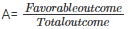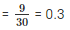2. Probability of a student having blood group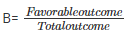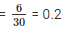3. Probability of a student having blood group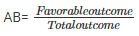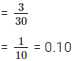4. Probability of a student having blood group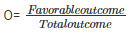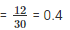Q9. Eleven bags of wheat flour, each marked 5kg, actually contained the following weights of flour (in Kg)

 4.97 5.05 5.08 5.03 5 5.06 5.08 4.98 5.04 5.07 5

Find the probability that any of these bags chosen at random contains more than 5 kg of flour.

Number of bags weighing more than 5kgs = 7

Total no of bags = 11

Probability of having more than 10kgs of rice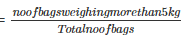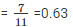Q10. The following table show the birth month of 40 students in class IX:

 JAN FEB MAR APR MAY JUNE JULY AUG SEP OCT NOV DEC 3 4 2 2 5 1 2 5 3 4 4 4

Find the probability that a student is born in October

1. Probability that a student is born in the month of October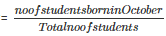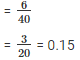Q11. Given below is the frequency distribution table regarding the concentration of SO2 in the air in parts per million of a certain city for 30 days.

 Concentration of SO2 0.00-0.04 0.04-0.08 0.08-0.12 0.12-0.16 0.16-0.20 0.20-0.24 No of days 4 8 9 2 4 3

Find the probability of the concentration of SO2 in the interval 0.12-0.16 on any of these days.

Total no of days: 30

Probability of concentration of SO2 in interval 0.12-0.16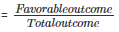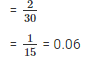Q12. A company selected 2400 families at random and surveys them to determine a relationship between income level and the number of vehicles in a home. The information gathered is listed below

VEHICLES PER FAMILY:

 Monthly Income 0 1 2 Above 2 Less than 7000 10 160 25 0 7000-10000 0 305 27 2 10000-13000 1 535 29 1 13000-16000 2 469 29 25 16000 above 1 579 82 88

If a family is chosen at random find the probability that the family is:

1. Earning Rs 10000 – 13000 per month and owning exactly 2 vehicles.

2. Earning Rs 16000 or more per month and owning exactly 1 vehicle.

3. Earning less than Rs 7000 per month and does not own any vehicle.

4. Earning Rs 13000 – 16000 per month and owning more than 2 vehicles.

5. Owning not more than 1 vehicle.

6.Owning at least one vehicle

1. The probability that the family is earning 10000-13000 and is having exactly 2 vehicles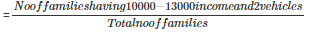= 292/400

2. The probability that the family is earning 16000 or more and is having exactly 1 vehicle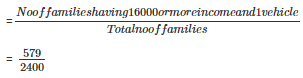3. The probability that the family is earning less than 7000 and is having no vehicle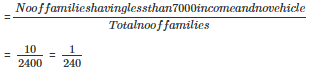4. The probability that the family is earning 13000-16000 and is having more than 2 vehicles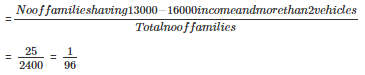5. The probability that the family is having not more than one vehicle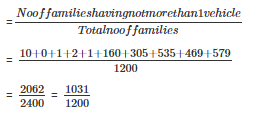6. The probability that the family is having atleast one vehicle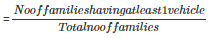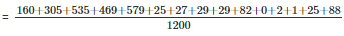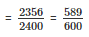Q13. The following table gives the life time of 400 neon lamps:

 Life time 300-400 400-500 500-600 600-700 700-800 800-900 900-1000 bulbs 14 56 60 86 74 62 48

A bulb is selected at random. Find the probability that the lifetime of a selected bulb:

1. Less than 400 hrs

2. between 300-800 hours

3. Atleast 700 hours

Total number of bulbs = 400

1. Probability that the life of the selected bulb is less than 400hrs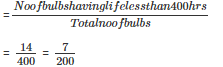2. Probability that the life of the selected bulb is between 300-800hrs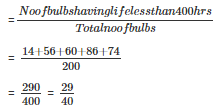3. Probability that the life of the selected bulb is atleast 700hrs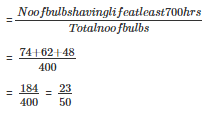Q14. Given below is the frequency distribution of wages (in Rs) of 30 workers in certain factory:

 Wages 110-130 130-150 150-170 170-190 190-210 210-230 230-250 No of workers 3 4 5 6 5 4 3

A worker is selected at random. Find the probability that his wages are:

1. Less than Rs.150

2. Atleast Rs.210

3. More than or equal to 150 but less than 210

Total number of workers = 30

1. Probability that the worker wages are less than Rs.150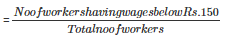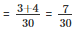2. Probability that the worker wages are atleast Rs.210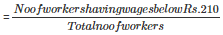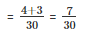3. Probability that the worker wages are more than or equal to 150 but less than 210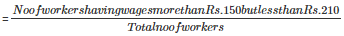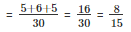Offer running on EduRev: Apply code STAYHOME200 to get INR 200 off on our premium plan EduRev Infinity!

91 docs

,

,

,

,

,

,

,

,

,

,

,

,

,

,

,

,

,

,

,

,

,

,

,

,

,

,

,

,

,

,

,

,

,

;